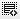Please explain liquidation preference in termsheets used by private equity funds0
Hi, I could not understand the maths behind the questions related to liqudating preferences. In one of the questions a VC has 15% equity in a company worth 100 million dollars with 2x as the liquidating preference. Company is sold for 2 billion dollars. How much money will the VC get? Answer was 470 million dollars. Not sure, of the answer. Please explain!0

Hi Gaurav, The math is as follows -
Sale Price = \$2 billion
VC gets 2x liquidation preference on the \$100mn investment = 2x100 = \$200mn
Cash left = \$2 billion - \$200mn = \$1.8 billion
VC will then get 15% of this \$1.8 billion = \$270mn
So total cash VC gets = \$200mn (from liq.pref) + \$270mn (from 15% equity) = \$470mn

Click on thisicon to add code snippet.